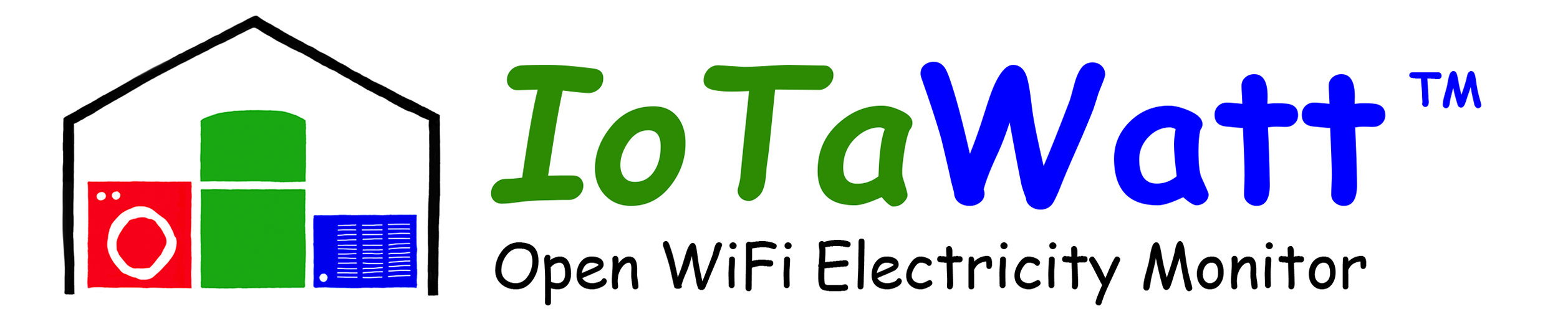# Incorrect CT configured

Can anyone advice what factor I can use to correct my measurement campaign data?
I have data measured using the white 200A clamps (ECS25200), but I only discovered after the 2 months of measuring that I configured the input as a (AcuCT-H100-200), I assume this is for this one. So I assume my data is not correct, but can be easily corrected by multiplying by a certain factor?
On the data specs, I can see they both have the same rated output of 50mA, but I don’t see any ‘turns’ specs?

The only difference between the two is the phase shift. The turns ratio is the same 200A:50mA. I don’t think any correction should be needed.

I installed 3 such clamps in a 3-phase system, what does the phase shift implies? Can there be an impact? My customer assumed a 3 times as high consumption than I measured/reported…

Every transformer, including the VT reference transformer, introduces a phase shift between the primary signal and the secondary. IoTaWatt uses tables to calculate the net shift between the VT and CT, and numerically shifts the VT signal to compensate. With ordinary loads at power factors above .60, the error without correction would typically be less than 1%.

If you have a three-phase install that is off by more than a couple of percent, it is most likely that the phases are not configured properly.

While the phase difference between VT and CT is typically in the 0-2 degree range, misconfigured three-phase is off by 120 degrees.

The effect of phase-shift on accuracy is roughly the cosine of the shift.

1 Like